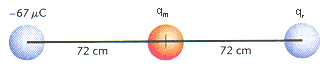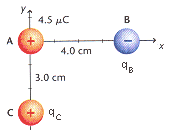Apr
01

# PreAP: Electrostatics 1 HW helps

Several folks have inquired about HW questions, particularly #’s 19-22.  These are certainly the more difficult problems on the HW assignment.  I will provide you with both some general information and some specific helps on each.

First, 19 & 20 are essentially the same.  19 has a North-South orientation, while 20 has an East-West orientation.  21 & 22 are similar except that you have more complicated 2-D vectors to consider.  Secondly, and perhaps the most important help I can give you: Ignore the signs when you use Coulomb’s Law.  Ignore the signs while you are doing your calculations and when you are done ask yourself, “What is the direction of this force?”  The remainder of the problem will depend on how you utilize that info.

Some background: If you keep the signs in the calculation when doing Coulomb’s Law Q1*Q2 give some interesting results.  If you have like charges (either + &+ or – & -) you will always end up with a positive force.  What does this mean?  Well, like charges repel, so a positive result must mean the charges push each other away.  Does the opposite hold?  Well if Q1 & Q2 have opposite signs (e.g., + & -) and you apply Coulomb’s Law your result will be negative.  This means an attractive force, or a force along a direction toward the second charge.

Let’s begin with the easier scenarios (19 & 20).

Three particles are placed in a line. The left particle has a charge of -67 µC, the middle, qm = +42 µC, and the right, qr = –87 µC. The middle particle is 72 cm from each of the others, as shown in Figure 20-13.Figure 20-13.

(a) Find the net force on the middle particle.
Let’s get some labels here.  Call the Left particle A, middle B, and right C.

In order to find the force on B we only need to calculate the force of A on B and of C on B.  The force of A on C is irrelevant to this problem.

So Fnet = FAB + FCB

FAB = ?    B is pulled to the LEFT

FCB = ?     B is pulled to the RIGHT

Since these forces are in opposite directions you need to treat them so, so when you calculate the net or total force you must SUBTRACT.

Part B)

Essentially the same idea, but a little different.  TO find the force on the right particle, you want

Fnet = FAC + FBC.

You already know the value of FBC from part A, but you must reconsider that the direction will be to the LEFT, relative to charge C.

FAC will need to be calculated.  It’s direction is repulsive, so to the RIGHT on charge C.  Again, subtraction will be called for.  A final caution: When finding the magnitude of FAC, be sure to consider the whole distance between particles A and C.

#’s 21 & 22:

We will look at number 21.  Like 19 and 20 you have a couple forces to calculate and consider.  The only difference here is that you must apply your rules for adding vectors at funky angles (find the x- and y-components for each vector, add them, find the resultant).  As always, one must consider direction whenever we “add” vectors.  Looking closely at one of your classmates versions of 21:

Three charged spheres are located at the positions shown in Figure 20-14. Find the total force on sphere B. (qB = -7.4 µC, and qC = +5.5 µC.)First use Coulomb’s Law to find the two forces: The force of A on B, and the force of C on B.  Both are attractive, so charge B is pulled generally toward the left.
When I solved with your numbers, I found the following vectors:
187 N to the Left
146 N @ 36.9 degrees South of West

Breaking into components and adding the x- and y-directions respectively I get:
X: -304 (left)
Y: -87.7 (South)

Using trig an Pythagorean, I conclude with: 316 N @ 16.1 degrees South of West (take care in putting that into Webassign as you need to refernce the whole angle from 0-degrees).